# for use with Matlab®Polynomial methods for systems, signals and control

The Polynomial Toolbox is a package for systems, signals and control analysis and design based on advanced polynomial methods. It consists of as many as 222 M-files in MATLAB code and is easy to use.

## Background

Polynomials and polynomial matrices play an important role in linear system theory. They do not only arise because polynomials cannot be avoided, but also because from first principles multivariable linear systems are modeled by sets of differential equations in the input u and the output y of the form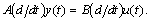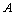where A and B are polynomial matrices in the differential operator d/dt (or the delay operator for discrete-time systems). Polynomial matrix models do not replace state space and frequency domain descriptions but provide a powerful additional tool.

## Polynomial Matrix Operations

To define polynomial matrices is as simple as typing

 » A=[1 1+s; 1-s 2*s]; » B=[s 0; 0 s];

It is even easier to compute with them

 » A+B ans = 1 + s   1 + s 1 - s      3s » A*B ans = s       s + s^2 s - s^2    2s^2 » det(A) ans = -1 + 2s + s^2

For larger matrices the Polynomial Matrix Editor is available.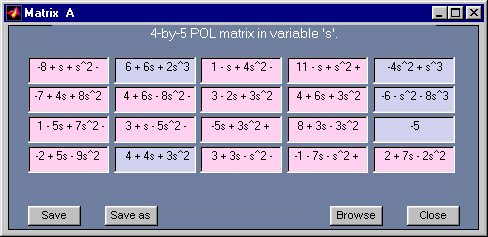Polynomial Matrix Editor

## Algorithms

The Polynomial Toolbox implements new original algorithms that are fast and reliable. This includes linear matrix polynomial equation solvers based on Sylvester matrices, the application of FFT for rank, determinant and other functions, a variety of new algorithms for spectral factorization and much more.

## Polynomial Matrix Fractions

The modeling of single-input single-output LTI systems often leads to differential equations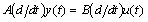with A and B polynomials or, equivalently, to the transfer function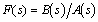of the system. For multi-input multi-output systems A and B become polynomial matrices and the transfer matrix is expressed in polynomial matrix fraction (PMF) form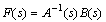The Polynomial Toolbox provides many macros for PMFs such as conversion between left and right fractions (lmf2rmf, rmf2lmf), properties testing (isprime, ispropper, isstable), and zero-pole plots (zpplot).

## Polynomial Equations

Feedback control system design by polynomial methods naturally introduces linear polynomial matrix equations such as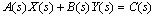where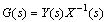is the controller transfer matrix. The Polynomial Toolbox offers numerous solvers for the equations suitably named axbyc, xaybc, axybc, and alike.

Next to the linear equations, optimum design problems naturally call for quadratic equations with polynomial matrices such as spectral, J-spectral or even nonsymmetrical factorizations (spf, spcof, fact ).

## Analysis

The Polynomial Toolbox offers simple programs for classical analysis. Its built-in convertors make all the tools of Control System Toolbox available for systems described by PMFs. In addition a wide range of macros is provided to test robustness of various kinds for systems with parametric uncertainties, including single parameter stability margins ( stabint), interval polynomials (kharit, khplot), and polytopic uncertainties (ptopplot, ptopex, etc.)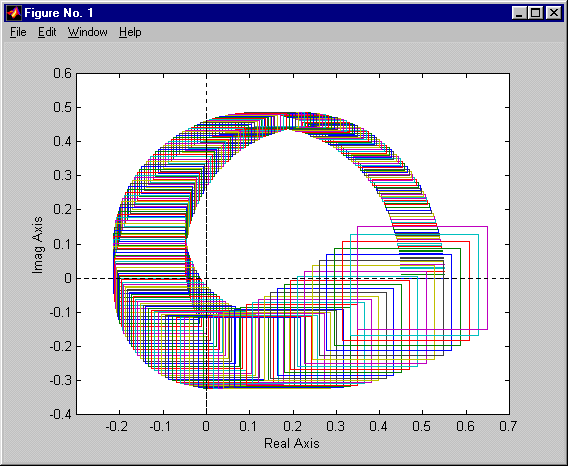Robust stability analysis for interval polynomials

## Design

Frequency domain solutions of many famous and proven design methods are directly provided, including

 plqg LQG design dsshinf sub- and optimal H-infinity design mixeds mixed sensitivity problem debe deadbeat control pplace pole placement stab all stabilizing controllers

Many other design routines can easily be developed based upon the basic polynomial matrix macros.

Numerous convertors enable direct cooperation with the Control System Toolbox

 lti2lmf, lti2rmf LTI objects to PMF ss, tf, zpk PMF to LTI objects lmf2dss, rmf2dss PMF to descriptor systems dss2lmf, dss2rmf descriptor systems to PMF

and the Symbolic Math Toolbox

 sym polynomial matrix to symbolic format sym2pol symbolic format to polynomial matrix

A SIMULINK 2 block set for LTI systems described by polynomial matrix fractions is also provided.

## Users

Users of the Polynomial Toolbox include control engineers involved in control systems analysis and design, communication engineers with an interest in filter design, and university teachers engaged in a variety of courses in linear systems, signals, and control.